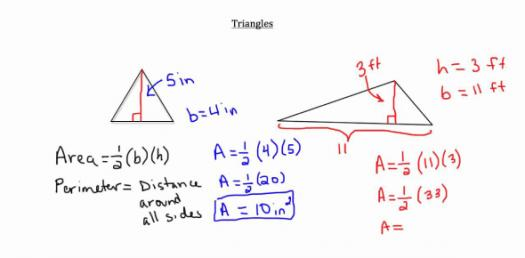# Quiz: Solve The Basic Geometry Questions

13 QuestionsSettings.

Related Topics
• 1.
Which formula is used to find area of a parallelogram?
• A.
• B.
• C.
• D.
• 2.
Which formula is used to find area of a circle?
• A.
• B.
• C.
• D.
• 3.
Which formula is used to find area of a triangle?
• A.
• B.
• C.
• D.
• 4.
Which formula is used to find area of a trapezoid?
• A.
• B.
• C.
• D.
• 5.
Which formula is used to find area of a rectangle?
• A.
• B.
• C.
• D.
• 6.
To find the area of a square, use the same formula you would use to find the area of a rectangle.
• A.

True

• B.

False

• 7.
• A.
• B.
• C.
• D.
• 8.
The students had a relay race on field day.  The race was arranged in a triangular formation.  It had a base of 25 yards and a height of 50 yards.  What was the area of the race field?
• A.
• B.
• C.
• D.
• 9.
The roof of a doghouse is shaped like a parallelogram.  The roof has a base of 4.5 feet and a height of 5.2 feet.  What is the area of the roof of the doghouse?
• A.
• B.
• C.
• D.
• 10.
Mrs. Porter is painting purple circles on the sidewalk with a diameter of 14 inches.  What is the area of the circles?
• A.
• B.
• C.
• D.
• 11.
James has a square rug that measures 12.6 feet on each side.  Find the area of the rug.
• A.
• B.
• C.
• D.
• 12.
Which formula is used to find area of a parallelogram?
• A.
• B.
• C.
• D.
• 13.
Find the area of a rectangle with a base of 2.5 cm and a height of 8.1 cm.
• A.
• B.
• C.
• D.